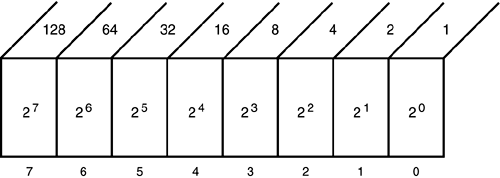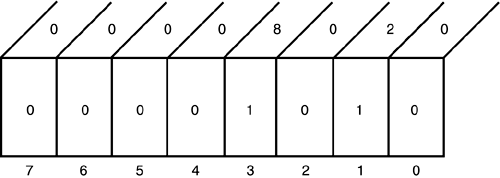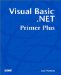# Binary Numbers

### Binary Numbers

Before you leave this chapter, you need to examine the binary numbering system in some detail. As mentioned in Chapter 2, a computer understands only two things: on and off. Because a computer understands only these two states, it interprets all values by using base-2, or binary, arithmetic. Figure 4.11 shows how a byte is interpreted using binary arithmetic.

##### Figure 4.11. The binary numbering system.In Figure 4.12 the numbers along the bottom correspond to the bit positions in an 8-bit byte of memory. When viewed this way, the rightmost bit is called the least significant bit and the leftmost bit is called the most significant bit. The numbers along the bottom simply number the bits, from most significant (that is, bit number 7) to least significant (that is, bit number 0), reading from left to right.

Each bit position has a numeric value that is equal to its bit position as a power of 2. For example, if the bit in bit position 0 is turned on, it has a value equal to 2 , which equals 1. If the bit in bit position 1 is turned on, its value is 2 1 , which equals 2. The upper row of numbers in Figure 4.11 shows the value for each bit position if that particular bit is turned on, expressed as a decimal number. Summing across all bit positions gives you the binary value for the memory byte. How does this work?

Suppose a byte in memory has the following bit pattern:

00001010

You can see that bits 1 and 3 are turned on; all the other bits are turned off. You can depict this bit pattern as shown in Figure 4.12.

##### Figure 4.12. Determining the value of bit pattern 00001010.If you look at the bits that are turned on and raise 2 to the bit position's power, you get the row of numbers seen near the top of Figure 4.12. In other words, you have

(2 7 x0) + (2 6 x0) + (2 5 x0) + (2 4 x0) + (2 3 x1) + (2 2 x0) + (2 1 x1) + (2 x0)

= 0 + 0 + 0 + 0 + 8 + 0 + 2 + 0

= 8 + 2

= 10

This shows that the bit pattern 00001010 is the binary representation for the decimal number 10. You should see that if all bits are turned on, 11111111 has a decimal value of 255. What would the value be if a "nibble" were turned on? That is, what is 00001111 in decimal arithmetic?

What if you need a number larger than 255? No problem. You add another 8 bits on the left side of Figure 4.12 and label its rightmost bit position 2 8 and continue leftward. The last bit position would be 2 15 , which equates to a value of 32,768. Now look at the range of values for a Short data type in Table 4.1. Do you see any relationship? (The value appears to be off by 1, but that's because of the way the sign bit is used in binary arithmetic.)

What happens if you slide the bits to the right one position so the bit pattern in Figure 4.12 becomes this:

00000101

You should find that the decimal value is 5. This is an old assembly language trick: Shifting the bits to the right one position divides the number by 2, and shifting the bits to the left one position multiplies by 2. Try it. This shouldn't be too surprising because we're using base-2 arithmetic. In decimal arithmetic, if you take a 1 and shift it one position to the left, it becomes 10. The left-shift by one position multiplies by the base being used. Take 100 and right-shift it one position, and it becomes 10, which is the same as dividing the number by 10. Just think how you will be able to amaze your friends with all this stuff at your next dinner party! Because the CPU instruction sets include bit shifting, which is a much faster operation than multiplying or dividing, bit shifting is a common compiler optimization trick.

Although you might not use binary arithmetic directly in your programming endeavors, it is important that you understand the basics of it. If nothing else, it should help you understand why the values presented in Table 4.1 have the values they do.Visual Basic .NET Primer Plus
ISBN: 0672324857
EAN: 2147483647
Year: 2003
Pages: 238
Authors: Jack Purdum

Similar book on Amazon